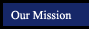Algebra 1 Concepts and Skills
Chapter 3
- Solving Linear Equations
 Section Title Tutorials from this book Examples to Copy (pop-up window) 3.1 Solving Equations Using Addition and Subtraction 25 33 39 43 47 49 53 a b c Practice Game -Solving 1-Step Equations Using Addition and Subtraction Practice Game -Solving 1-Step Equations Using Addition and Subtraction (With a Fraction!) Practice Game -Solving 1-Step Equations Using Addition and Subtraction (With 2 Fractions!) 3.2 Solving Equations Using Multiplication and Division 23 27 29 31 33 35 37 51 a b c d e Practice Game -Solving 1-Step Equations Using Multiplication and Division f Practice Game - Solving 1-Step Equations with Fractions Next to the Variable 1 Step Equation Practice Activiity (random mix of addition, subtraction, multiplication and division) 3.3 Solving Multi-Step Equations 23 25 27 29 31 33 35 37 39 a b c d e 2 Step Equation Practice Game - Level 0 2 Step Equation Practice Game - Level 1 f g h i 3.4 Solving Equations with Variables on Both Sides 21 23 27 33 39 Practice Game - Equations With Variables on Both Sides - Level 0 Practice Game - Equations With Variables on Both Sides - Level 1 Practice Game - Equations With Variables on Both Sides - Level 2 3.5 More on Linear Equations 19 21 27 29 31 33 Practice Game - Variables on Both Sides, With Parentheses Practice Game - Variables and Parentheses on Both Sides - Level 0 Practice Game - Variables and Parentheses on Both Sides - Level 1 3.6 Solving Decimal Equations 23 25 27 29 31 33 3.7 Formulas 11 13 15 17 21 3.8 Ratios and Rates 15 17 19 21 23 3.9 Percents 13 15 17 19 21 23 25 29 37 39
 Suggested Assignments Section Topic Page Exercises 3.1 Solving Equations Using Addition and Subtraction P135-137 x 19 – 59 + 3 “examples to copy” 3.2 Solving Equations Using Multiplication and Division P141-143 x16 – 52 + 3 “examples to copy” 3.3 Solving Multi-Step Equations P147-148 x19 – 46 + 3 “examples to copy” Quiz 1 P149 x1-15 show work and check to receive credit 3.4 Solving Equations with Variables on Both Sides P154-156 x17 – 48 + 3 “examples to copy” 3.5 More on Linear Equations P160-162 x19 – 40 + 3 “examples to copy” 3.6 Solving Decimal Equations P166-168 x20 – 36 + 3 “examples to copy” Quiz 2 P169 x1-22 show work and check to receive credit 3.7 Formulas P174-176 x11- 21 + 3 “examples to copy” 3.8 Ratios and Rates P180-182 see contract 3.9 Percents P186-188 see contract Quiz 3 P188 x1-9 Chapter Review P189-191 x1-25 show work and check to receive credit Chapter Test P193 x1 - 23

Notes for sections from AlgebraNotes.com

Online Scientific Calculator from Calculator.com

Graphing Calculator by CoolMath.com

Math Glossary (more sophisticated than it looks!)